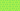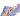PricingResources

# What is econometrics?

Written by

The term “econometrics” was first coined by Polish economist Pawel Ciompa in 1910. However, it was Ragnar Frisch and Jan Tinberg who defined its modern use and meaning, earning them the Nobel Prize in Economics in 1969. Here’s a look at how econometrics methods are put into use today.

## Understanding econometrics for finance

Econometrics uses a blend of statistical and mathematical methods to test theories and predict future economic trends. With a blend of statistical inference, economic theory, and basic mathematical principles, econometrics for finance helps describe modern economic systems.

Essentially, it turns qualitative ideas into quantitative outcomes. For example, econometrics analysis can be used to transform a theoretic model into an actual tool or outcome that policymakers can put into practice. To do this, econometricians are the ones who sift through massive piles of data, distilling it into quantitative statements using modeling and analysis.

## Econometrics vs. statistics

Although there is some overlap when it comes to econometrics vs. statistics, the two terms are different in meaning. Econometrics does use statistical theories and data in its analysis of economic theories, but it involves more than just numbers. It looks at the bigger picture of economics when applying statistical methods.

There are still many similarities in the study of econometrics vs. statistics. To perform an econometrics analysis, an economist might use statistical tools, including:

• Probability

• Frequency distributions

• Statistical inference

• Correlation analysis

• Time-series methods

## What is the purpose of econometrics?

An econometrics analysis is used to test a hypothesis, whether it’s an existing economic theory or a brand new idea. It can also be used to forecast future financial or economic trends using current data. This makes econometrics for finance an everyday tool for Wall Street traders and financial analysts.

The idea behind econometrics can be applied to test many theories. For example, an economist might want to test the hypothesis that as a company increases its profit, its spending will increase accordingly. Econometrics would take the data behind this assumption and then use statistical tools like regression analysis to look more deeply into the relationship between profit and spending.

## Econometrics basics: theoretical vs. applied

There are two primary components of econometrics: theoretical and applied. Here’s a little bit more information about how these elements of econometrics for finance work.

### Theoretical econometrics

This brand of econometrics analysis looks at the properties of existing statistical procedures or tests to estimate any unknowns. Theoretical econometricians might develop new statistical methodologies that account for anomalies in economic data. This branch of econometrics relies primarily on theoretical statistics, numerical data, and mathematics, with the aim of proving that new procedures are indeed viable.

### Applied econometrics

The second component of econometrics uses techniques to transform qualitative statements into quantitative statements. When theoretical econometricians develop new statistical procedures, it’s often in response to the work of applied econometricians who have discovered unexplained variances in data sets. The applied econometricians can then use these new techniques to test their hypotheses.

## Econometrics methods

There are a couple of steps involved in standard econometrics methods. The very first step is to choose a set of data for analysis. This could be anything from inflation rates to unemployment figures or historical fintech stock prices.

1. With the data selected, an econometrist suggests a hypothesis or theory to explain it. This model should define the different variables involved, along with the magnitude of the relationship between the variables. Economic theory plays a large role in this initial stage of econometrics analysis.

2. The next step is to specify a statistical model that best relates to the economic theory being tested. A linear relationship is usually assumed, which means that any change in explanatory variables will produce the same level of change in a dependent variable for a straight-line progression.

3. You’ll then use a statistical procedure to estimate any unknown parameters or coefficients of the model. Econometrics software usually makes short work of this step.

4. The hypothesis should be analyzed logically to see if it makes sense within the estimated parameters and economic theories.

5. Finally, it’s time to test the hypothesis to see if the estimated parameter holds.

It’s important to remember that although econometrics methods can yield accurate forecasts, tools like regression analysis alone aren’t enough to prove causation. For example, two data sets might appear to be associated, but this cannot be confirmed without an adequate causal theory.

## We can help

GoCardless helps you automate payment collection, cutting down on the amount of admin your team needs to deal with when chasing invoices. Find out how GoCardless can help you with ad hoc payments or recurring payments.## Interested in automating the way you get paid? GoCardless can help

Contact sales

Sales

Contact Sales

+1(415) 523-2279

Support

help@gocardless.com

+1 (628) 241-0044